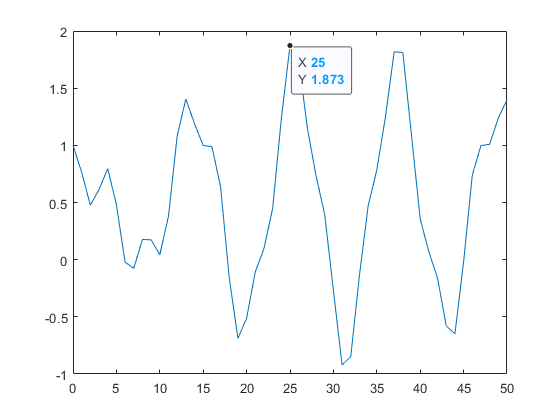## File Exchange Pick of the WeekOur best user submissions

Richard is Consulting Engineer at MathWorks focused on the Embedded Coder product for code generation, primarily in the Aerospace industry.

Richard’s pick this week is add_Data_Tips by Massimo Ciacci.

### Contents

#### Pick

My pick this week is the utility function add_Data_Tips for programmatically adding data tips to multiple lines in a plot.

If you write custom scripts/functions to plot data it is often beneficial to highlight specific points in the plot such as minimums/maximums. Datatips allow you to show the values of the data at selected locations in the figure.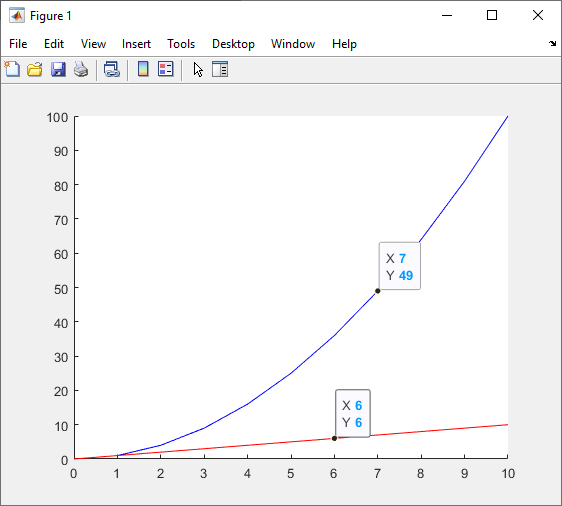While you can add data tips to a plot manually, it is difficult to add data tips to multiple lines at the same location on the X-axis. add_Data_Tips solves this problem. Using add_Data_Tips, you can insert data tips on multiple lines in a plot at a specified X-axis locations. For instance, if you want to call out the maximum value of a plot, you can use the max function to find the index of the maximum value of the Y-Axis data and specify the corresponding X-Axis data in the call to add_Data_Tips to insert a data tip in the figure at the maximum point.

n = 0:50;
y = cos(.5*(n)).^3 + sin(.3*(n)).^2;
figure
yh = plot(n, y);
[~,indx] = max(y);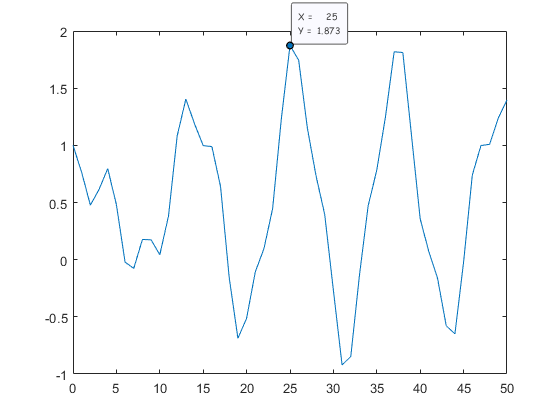If you have a figure with multiple plots, you can add data tips to any or all of the lines at the same X-Axis location by passing in the handles to the desired lines.

n = 0:50;
y = cos(.5*(n)).^3 + sin(.3*(n)).^2;
figure
yh(1) = plot(n, y);
hold on
y2 = sin(1.5*(n)).^3 - cos(1.3*(n)).^2;
yh(2) = plot(n,y2);
[~,indx] = max(y);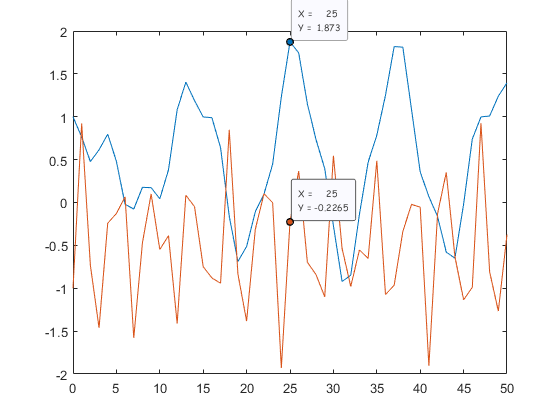If you already have a plot, you can get the handles to the lines by accessing the Children of the axes

CurrentAxes = gca;
LinesHandles = CurrentAxes.Children;
x_location = 10;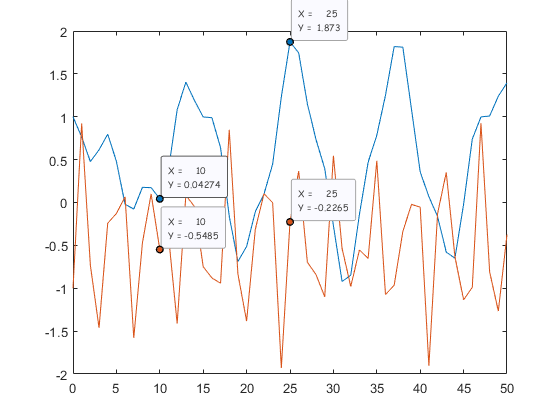You can use the examples above or the File Exchange submission includes a demo script, ‘test_add_DataTips.m’, that provides an example.

#### New in R2019b

If you have access to the most recent release, R2019b, you can now programmatically create datatips using the new datatip function.

figure
yh = plot(n, y);
[~,indx] = max(y);
datatip(yh,'DataIndex', indx);